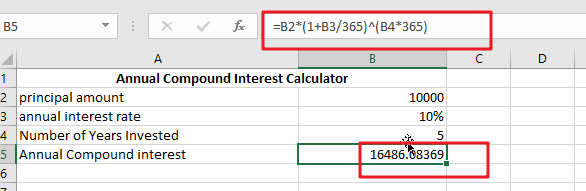# Calculate Compound Interest in Excel

The post shows how to determine the future value of an investment using the annual compound interest schedule formula in Excel and includes examples of how to do it using yearly, monthly, or daily compounding interest rates. Additionally, you’ll get thorough instructions for creating your own Excel compound interest calculator.

Compound interest is a fundamental tenet of finance and one of the most powerful financial forces at work, determining the result of your investments.

Unless you are a graduate of accounting, a financial analyst, or an experienced investor, it may be difficult to comprehend the notion included in specialist financial books and manuals. The purpose of this essay is to simplify. Additionally, you will learn how to calculate Compound Interest in Excel.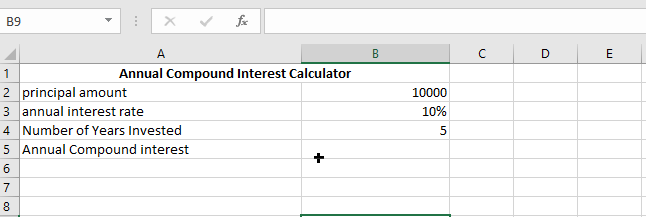## What is compound interest?

Compound interest, in the simplest definition, is interest gained on interest. Compound interest is generated on both the original investment (principal) and the interest collected over time.

Perhaps it is simpler to begin with basic interest, which is computed on the principal amount alone.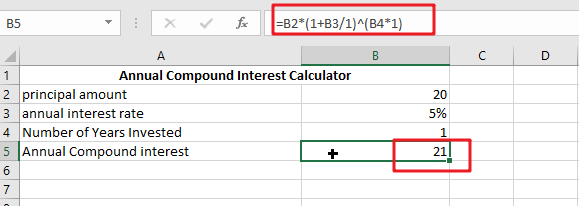For example, suppose you deposit \$20 into a bank account. How much would your deposit be worth after a year at a rate of 5% yearly interest? It should be 21.4. (20 + 20*0.05 = 21), and your interest earned is \$1.

In the case of compound interest, the principle is different for each time period. The bank will not return the interest earned; instead, it will be added to your main investment. This increased principle amount becomes the principal for the next time period and earns interest as well. you earn interest on both the principle and the interest gained during each compounding period.

In our case, in addition to the \$20 capital, the \$1 earned interest will accrue interest the next year. Thus, how much would your \$20 investment be worth after two years at a compounded annual interest rate of 5%? It is 22.05 (21 + 21*0.05 = 22.05), and your interest earned is \$2.05.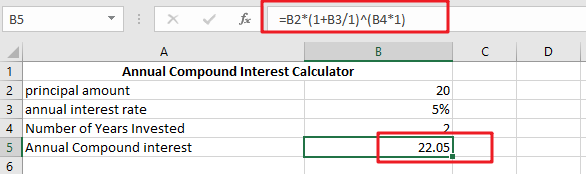There are various methods for calculating compound interest in Excel, and we will cover each in depth.

## Compound interest calculation in Excel

Long-term investing may be an efficient way to build wealth, and even tiny amounts can add up over time. The following Excel compound interest calculations will assist you in putting the savings plan into action. Eventually, we’ll develop an uniform method for calculating the future value for various compounding periods – daily, weekly, monthly, quarterly, or annual.

### Formula for Compound Interest in General (for Daily, Weekly, Monthly, and Yearly Compounding)

In Excel, a more efficient method of computing compound interest is to use the general interest formula:

`FV = PV(1+r)^n`

Where FV denotes future value, PV is current value, r denotes the period-to-period interest rate, and n denotes the number of compounding periods.

Consider investing \$5,000 at a 10% annual interest rate compounded semi-annually and calculating the worth of your investment after five years. The spreadsheet below demonstrates how to do this computation in Excel.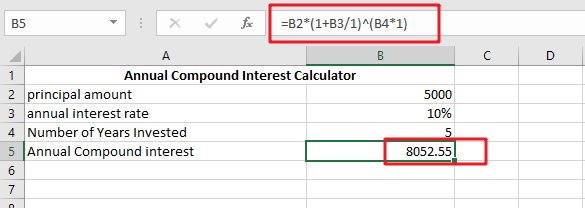### Calculating annual compound interest in Excel

To help you grasp the concept of compound interest, let’s revisit the very basic example given at the start of this course and create an Excel formula to compute yearly compound interest. Assume that you are investing \$10000 at a 10% annual interest rate and are curious in how annual compounding boosts your money.### Annual Compound Interest Calculator

To calculate annual compound interest, just add the previous year’s interest to the next year’s principle amount.

Additionally, the excel formula for annual compound interest will be included.

`=Principal Amount*((1+Annual Interest Rate/1)(Number of Years Invested*1)))`

The first year, you will get \$10000*10 percent, which equals \$1000; the second year, you will receive (\$10000+\$1000)*10 percent, which is \$1100; and so on. After 5 years, you should receive about \$16105.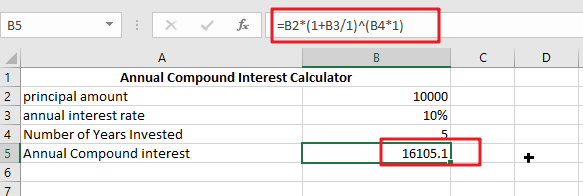### Formula for Quarterly Compound Interest

Calculating compound interest on a quarterly basis is identical to calculating compound interest on an annual basis.

However, in this case, interest must be calculated four times a year.

Each quarter’s interest will be added to the next quarter’s principal.

To compute quarterly compound interest, use the formula below.

`=Principal Amount*((1+Annual Interest Rate/4)(Number of Years Invested*4)))`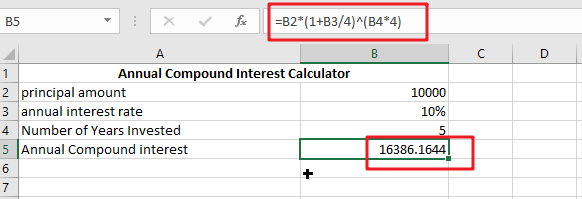With a Principal amount of \$10000 and a ten percent interest rate for five years, we will get \$16386.

In the first quarter, we earn 10000*(10% /4) = \$250; in the second quarter, we get (\$10000+\$250)*(10% /4) = \$256; and so on for twenty quarters (5 years).

### Formula for Monthly Compound Interest

When computing compound interest on a monthly basis, you must apply the same premise as you used for prior time periods.

At the conclusion of each month, you must compute the interest. Additionally, this approach divides the interest rate by 12 to get a monthly interest rate.

In Excel, you can use the following formula to compute monthly compound interest.

`=Principal Amount*((1+Annual Interest Rate/12)(Total Years of Investing*12))))`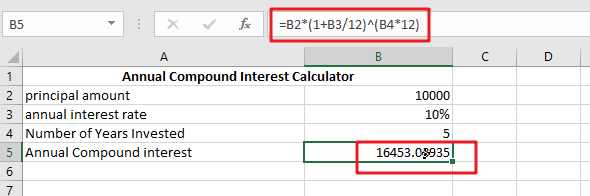With a principle amount of \$10000 and a ten percent interest rate for five years, we will get \$16453.

In the first month, we get 10000*(10% /12), which equals \$83.33, and in the second month, we receive (\$10000+\$83.33)*(10% /12), which is \$84.02, and so on for 60 months (5 years).

### Formula for Daily Compound Interest

Again, we must utilize the same procedure using the following calculation formula for computing daily compound interest.

Divide the interest rate by 365 to get the daily interest rate. Thus, you may compute daily compound interest using the method below.

`=Principal Amount*((1+Annual Interest Rate/365)(Total Years of Investing*365)))`With a principle amount of \$10000 and a ten percent interest rate for five years, we will get \$16486.

On the first day, we receive 10000*(10% /365), which equals \$4; on the second day, we get (\$10000+\$4)*(10% /365), which equals \$4; and so on for the next five years.